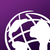# Support for 3D methods and M values on ArcPy geometries

597
1
02-24-2016 03:43 AM
Status: Open
Labels (2)byEsri Esteemed Contributor
Currently (ArcMap 10.3.1 and probably 10.4 too, ArcGIS Pro 1.2) the methods on the arcpy geometries do not support 3D or M values. For instance the method to determine a position on a line will do this 2D and not take into account the third dimension, if the geometry has it:

arcpy.Polyline()::positionAlongLine (value, {use_percentage})

See discussion: https://geonet.esri.com/message/589921

Neither is it possible to extract a part of a polyline based on the M values with

arcpy.Polyline()::segmentAlongLine (start_measure, end_measure, {use_percentage})

... since the measures mentioned as parameters are distances (absolute or in percentage of the total length of the polyline).

See discussion: https://geonet.esri.com/message/516789

My request is to add additional methods and properties to ArcPy geometries to support Z and M values.
Perhaps initially additional methods for using M values for:
arcpy.Polyline()::segmentAlongLineM(start_measure, end_measure, {use_percentage})
arcpy.Polyline()::positionAlongLineM(value, {use_percentage})

Additional methods for using 3D length values for:
arcpy.Polyline()::segmentAlongLine3D(start_measure, end_measure, {use_percentage})
arcpy.Polyline()::positionAlongLine3D(value, {use_percentage})
arcpy.Polyline()::getLength3D({measurement_type}, {units})

... but I can imagine that these would be helpful too:
buffer3D(distance)
distanceTo3D(other)
measureOnLineM(in_point, {as_percentage})
measureOnLine3D(in_point, {as_percentage})
pointFromAngleAndDistance3D(angle, distance, {method})
queryPointAndDistance3D(in_point, {as_percentage})

... and others

1 CommentHaving 3D full stop would be nice. Imagine flaythroughs, fast 3D rendering, 3D measuring, navigation and ability to create 3D webservices on ArcServer without Portal. Pity Google isnt interested in GIS.